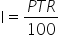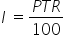Mathematics
Easy

Question

# Simple interest is given by. If p = \$1000, T = 3 years and R = 5%. Find the simple interest.

## 150    200    300    400Hint:

## The correct answer is: 150

### The given expressions isThe value assigned are as follows:P = \$1000T = 3 yearsR = 5%We are asked to find the value of simple interest or ‘I’.To substitute the values, we will replace the variables by their values.After substituting the values, we will perform the required operations.So, the value of simple interest is 150.

We have to be careful about the operations because of the variables. We have to follow the proper procedure for performing the operations. The variables take different values under different conditions. So we have to be careful while substituting the values. The main part of this type of question is just replacing the variables by their values and performing the operation.

### Related Questions to study#### With Turito Foundation.#### Get an Expert Advice From Turito.